This tutorial is about how to make Meshes dissolve in the pattern of a texture. We will use a surface shader for this tutorial, so if you don’t understand how they work yet, I can refer you to my tutorial about them here. This tutorial will also just work with opaque shaders, but you can also use the same principles on transparent or unlit shaders.

## Simple Dissolve

We start by adding a new texture to our shader to drive the dissolve. We’ll simply use the uv coordinates and then write the result to the albedo channel. If our model doesn’t let us do that we can also use different UV coordinates, for example screenspace or triplanar ones or even generate the data procedurally.

From the read value we’ll only use the red channel. Any single channel is fine, the red one just happens to be the first. If you use several things that use a single texture channel you can also use different channels of a single texture.

``````//in properties

_DissolveTex ("Dissolve Texture", 2D) = "black" {}
``````
``````// in CGPROGRAM with other shader variables

sampler2D _DissolveTex;
``````
``````// in input struct
float2 uv_DissolveTex;
``````
``````// in surface function

float dissolve = tex2D(_DissolveTex, i.uv_DissolveTex).r;

o.Albedo = dissolve;
``````

The dissolve texture I’m using is a perlin texture I baked via the material baking tool I explained in a previous tutorial.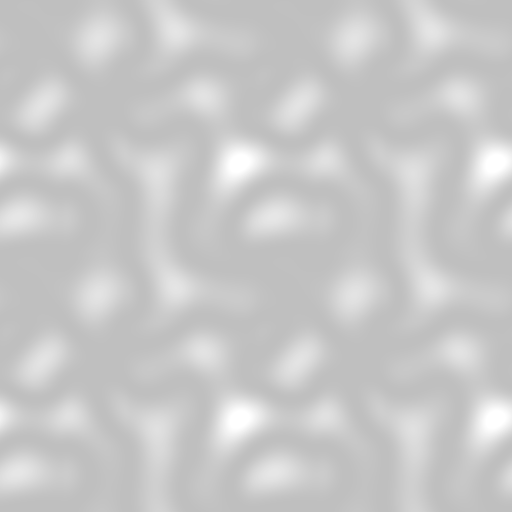When outputting the texture as the albedo channel, the model should look approximately like this: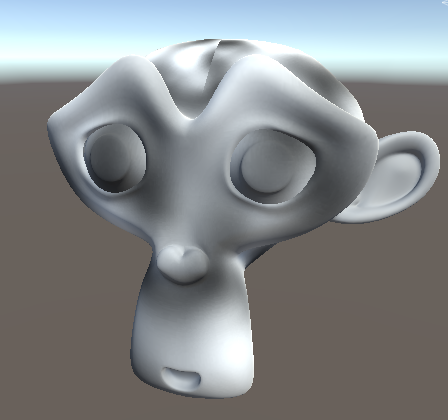The next step is to make the model disappear depending on a dissolve value. For this we use the clip function. When we pass a negative value to it the shader stops calculations and doesn’t render the pixel. If we pass it a positive value or zero the function does nothing. So to make the black areas of out dissolve texture to disappear first, we subtract the dissolve value from the dissolve pattern. With a dissolve value of zero there won’t be any negative values, so the model will render as usual. When we increase the dissolve value, all the pixels where the texture value is less than the dissove variable the result of the subtraction will be negative and the model won’t render until the dissolve value is at 1 where only pixels will be shown where the texture is white. To stop white pixels from always showing we can multiply the texture value by a number close to 1, but a bit less, so it never reaches 1.

``````// in properties

_DissolveAmount ("Dissolve Amount", Range(0, 1)) = 0.5
``````
``````// in CGPROGRAM with other shader variables

float _DissolveAmount;
``````
``````// in surface method

float dissolve = tex2D(_DissolveTex, i.uv_DissolveTex).r;
dissolve = dissolve * 0.999;
float isVisible = dissolve - _DissolveAmount;
clip(isVisible);
``````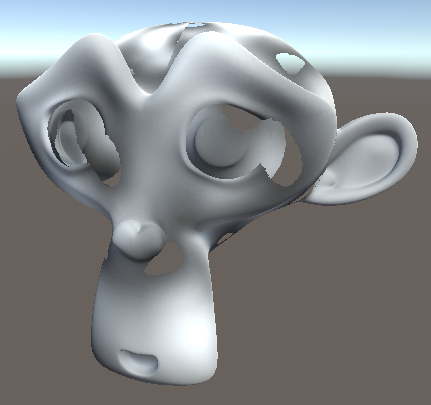Now the last fix is to stop outputting the dissolve texture to the albedo channel and instead use the color texture we used previously. Depending on your shader, the whole surface function could look something like this.

``````void surf (Input i, inout SurfaceOutputStandard o) {
float dissolve = tex2D(_DissolveTex, i.uv_DissolveTex).r;
dissolve = dissolve * 0.999;
float isVisible = dissolve - _DissolveAmount;
clip(isVisible);

fixed4 col = tex2D(_MainTex, i.uv_MainTex);
col *= _Color;

o.Albedo = dissolve;
o.Metallic = _Metallic;
o.Smoothness = _Smoothness;
o.Emission = _Emission;
}
``````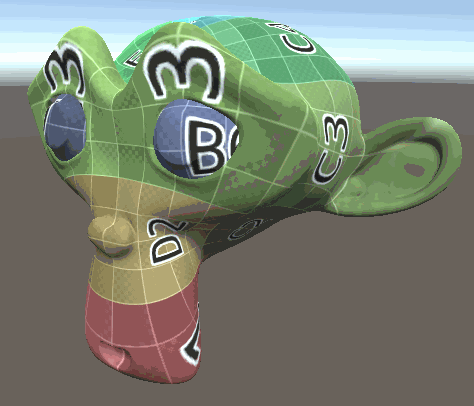## Dissolve Highlight

Because the `isVisible` variable slowly approaches zero, we can predict when we’re about to discard a pixel. A common effect is to make that border, just before we discard the pixel, glow. For that we’ll define a glow color, glow range and glow falloff. If you want to you can also use different metrics to decorate the border, including using a completely customisable texture like we did in the custom lighting tutorial.

``````//in properties

[HDR]_GlowColor("Color", Color) = (1, 1, 1, 1)
_GlowRange("Range", Range(0, .5)) = 0.1
_GlowFalloff("Falloff", Range(0, 1)) = 0.1
``````
``````// in CGPROGRAM with other shader variables

float3 _GlowColor;
float _GlowRange;
float _GlowFalloff;
``````

When we have those variables we can define where the glow should appear by doing a smoothstep. Because we want the lower values of the `isVisible` variable to map to higher visibility of the glow (0 is always 100% glowing and higher numbers mean further away from the cutoff) we first pass the glowrange plus the falloff, as a second parameter we pass just the glow range andthe third parameter is the one we evaluate, the `isVisible` variable itself. By doing this everything that’s closer to the cutoff than `_GlowRange` is completely glowing and everything that’s between `_GlowRange` and `_GlowRange` + `_GlowFalloff` has a gradient. We then multiply the variable that defines wether the pixel is glowing or not by the glow color and add it to the base emissive value of the shader.

``````// in surface method

float isGlowing = smoothstep(_GlowRange + _GlowFalloff, _GlowRange, isVisible);
float3 glow = isGlowing * _GlowColor;

o.Emission = _Emission + glow;
``````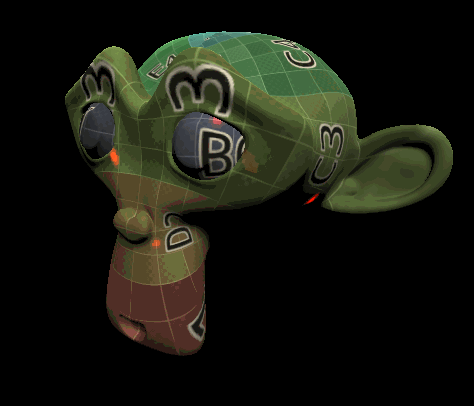## Source

``````Shader "Tutorial/038_dissolve" {
Properties {
_Color ("Tint", Color) = (0, 0, 0, 1)
_MainTex ("Texture", 2D) = "white" {}
_Smoothness ("Smoothness", Range(0, 1)) = 0
_Metallic ("Metalness", Range(0, 1)) = 0
[HDR] _Emission ("Emission", color) = (0,0,0)

_DissolveTex ("Dissolve Texture", 2D) = "black" {}
_DissolveAmount ("Dissolve Amount", Range(0, 1)) = 0.5

[HDR]_GlowColor("Color", Color) = (1, 1, 1, 1)
_GlowRange("Range", Range(0, .3)) = 0.1
_GlowFalloff("Falloff", Range(0.001, .3)) = 0.1
}
Tags{ "RenderType"="Opaque" "Queue"="Geometry"}

CGPROGRAM

#pragma target 3.0

sampler2D _MainTex;
fixed4 _Color;

half _Smoothness;
half _Metallic;
half3 _Emission;

sampler2D _DissolveTex;
float _DissolveAmount;

float3 _GlowColor;
float _GlowRange;
float _GlowFalloff;

struct Input {
float2 uv_MainTex;
float2 uv_DissolveTex;
};

void surf (Input i, inout SurfaceOutputStandard o) {
float dissolve = tex2D(_DissolveTex, i.uv_DissolveTex).r;
dissolve = dissolve * 0.999;
float isVisible = dissolve - _DissolveAmount;
clip(isVisible);

float isGlowing = smoothstep(_GlowRange + _GlowFalloff, _GlowRange, isVisible);
float3 glow = isGlowing * _GlowColor;

fixed4 col = tex2D(_MainTex, i.uv_MainTex);
col *= _Color;

o.Albedo = col;
o.Metallic = _Metallic;
o.Smoothness = _Smoothness;
o.Emission = _Emission + glow;
}
ENDCG
}
FallBack "Standard"
}
``````

Now you know how to make meshes dissolve into nothing with shaders! The next tutorial will probably be about signed distance fields again so stay tuned for that.

You can also find me on twitter at @totallyRonja. If you liked my tutorial and want to support me you can do that on Patreon (patreon.com/RonjaTutorials) or Ko-Fi (ko-fi.com/RonjaTutorials).